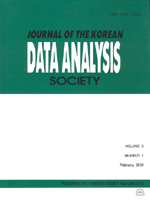상세검색
최근 검색어 전체 삭제
다국어입력
즐겨찾기0KCI등재 학술저널

# A Nonparametric Test under the Proportional Hazards Model

• 등재여부 : KCI등재
• 2016.08
• 1751 - 1757 (7 pages)

In this research, we propose a nonparametric test procedure under the proportional hazards assumption for the two-sample problem setting. For this, first of all, we review the functional equation for relative risk between the survival and cumulative functions under the proportional hazards model and the estimate of the relative risk proposed by Park, Hong (2007) with the expressions of the empirical ones for the computational convenience. Then we propose a nonparametric test procedure with the null distribution for the test statistic. For obtaining the null distribution, we apply the large sample approximation theorem and derive the asymptotic normality. Then we compare the performance of our proposed test with that of Begun (1987) with the exponential and Weibull distributions, which are frequently applied in the survival analysis by varying sample sizes. We show that our proposed test procedure can be an excellent alternative to the Begun’s one. Finally we discuss some interesting features of our procedure including re-sampling methods to obtain the null distributions of the test statistics as concluding remarks.

1. Introduction

2. A Review about Functional Equation and a Nonparametric Test

3. A Simulation Study

4. Some Concluding Remarks

References